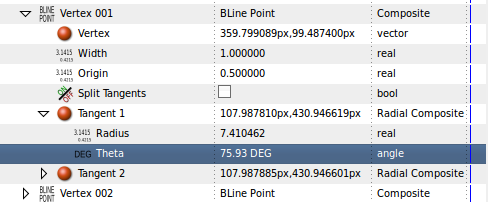# Tangent Radius , Theta , X and Y ??

im guessing this question goes in the coding area??

i just need to know: how does synfig figure out the tangent’s “x” and “y” pixels, using the “radius” and "theta "in the screenshot you can see that synfig has created the “x” and “y” pixels for “Tanget 1” by using the “radius” and "theta ".

the reason i ask is because synfig’s .sif/.xml doesent contain the “x” and “y” pixel values for tangents only the “radius” and “theta” (obviously synfig figures out the "x"and “y” values on the fly) , however i need to retrieve the “x” and “y” values to use in another apllication.

Im not a powerful 2d vector mathematician , if some one could just give me the formula that would be great.

[size=150]edit:[/size]
my math skills arent that good, but here goes

basically
each real number under “radius” is equal to 60px .
so if radius is equal to 1.00000 then that translates as 60px
if radius is equal to 0.50000 then that translates as 30px
radius in the screenshot is 7.410462
therefore radius is actually: 7.410462 x 60px = 444.62772px

the value of x (i think) is figured out as
cos(theta) x (radius x 60px)

0.2431071546 x 444.62772px = 108.0921798723px

its the closest i can get to 107.987810, (108.0 and 107.9 arent that far away)

the solution to the value of y( i think) is figured out as
sin(theta) x (radius x 60px)
0.9699994389 x 444.62772px = 431.2886389194px

its the closest i can get to 430.946601, (431.2 and 430.9 are quite far away )

im assuming synfig offsets numbers slightly to prevent computational errors

any help would be nice

Your maths are correct. Don’t bother on the precision. You only see partially the internal number so not try to calculate them.

Tangents are well managed in synfig using radius and angle. You can convert a tangent to a Composite (x,y composite) by right click on the tangent parameter and select Convert ->Composite. Notice that you can convert them again to Radial Composite to the form radius, angle. If the tangent is converted to x,y composite you cannot manipulate it using the ducks in the screen, because the program has not been coded for that.

So the best way to obtain the x and y values (maintaining the tangent as radius, angle is by following those steps:

1. Right click on the Radius parameter and select “Export”. Give it a name: “r”.
2. Repeat the same for the Theta. Name it “a”.
3. Those exportations allows to access to those parameters from any other parameter from other layer. Go to the CHILD panel and expand ValueNode triangle. Do you see them there?. Nice.
4. Now create one circle. (It can be any other layer that has a vector (x,y) as parameter. Select its Origin parameter. Right click on the Origin parameter and select Convert->Composite. Expand it and you’ll see the X and Y components.
5. Select the X component. Right click Convert->Cosine. Now it shows two parameters: Angle and Amplitude. Now select the “a” exported parameter in the CHILD layer. It will remain selected. Then select the Angle parameter and right click it. Select Connect. Now the Angle is linked to the “a” exported value that is exactly the same value than the exported Theta from the tangent.
6. Now select the “r” exported parameter in the CHILD panel and select the Amplitude parameter form the Cosine conversion. Right click and select Connect. Same comments but for Radius.
7. Repeat same steps with Y but use Sine convert instead Cosine convert.

Now if you manipulate the tangent duck that you exported its components you can see how the origin of the circle follows its turns. It turns around the (0,0).

You should know one thing: the drawn tangent duck is not a the same scale than the radius value. It is drawn at 1/3 of its radius. It is done in this way to avoid tangents to overlap one over other in a normal bilne.

Need more maths? Look here: synfig.org/Convert

-G

thanks Genete, that was big help.

i guess synfig is really a mathematics engine disguised as an animation studio.

Correct. It did start life out as sinfg, a chaos/mandelbrot set program:

sinfg.sf.net